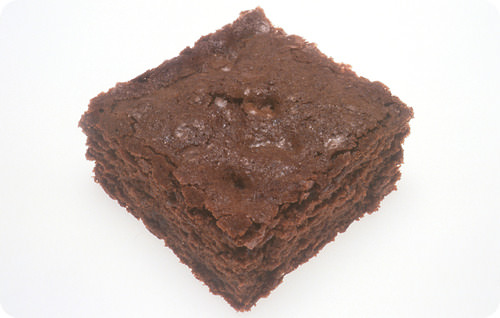# 12.8: Determining the Limiting Reactant

$$\newcommand{\vecs}{\overset { \rightharpoonup} {\mathbf{#1}} }$$ $$\newcommand{\vecd}{\overset{-\!-\!\rightharpoonup}{\vphantom{a}\smash {#1}}}$$$$\newcommand{\id}{\mathrm{id}}$$ $$\newcommand{\Span}{\mathrm{span}}$$ $$\newcommand{\kernel}{\mathrm{null}\,}$$ $$\newcommand{\range}{\mathrm{range}\,}$$ $$\newcommand{\RealPart}{\mathrm{Re}}$$ $$\newcommand{\ImaginaryPart}{\mathrm{Im}}$$ $$\newcommand{\Argument}{\mathrm{Arg}}$$ $$\newcommand{\norm}{\| #1 \|}$$ $$\newcommand{\inner}{\langle #1, #2 \rangle}$$ $$\newcommand{\Span}{\mathrm{span}}$$ $$\newcommand{\id}{\mathrm{id}}$$ $$\newcommand{\Span}{\mathrm{span}}$$ $$\newcommand{\kernel}{\mathrm{null}\,}$$ $$\newcommand{\range}{\mathrm{range}\,}$$ $$\newcommand{\RealPart}{\mathrm{Re}}$$ $$\newcommand{\ImaginaryPart}{\mathrm{Im}}$$ $$\newcommand{\Argument}{\mathrm{Arg}}$$ $$\newcommand{\norm}{\| #1 \|}$$ $$\newcommand{\inner}{\langle #1, #2 \rangle}$$ $$\newcommand{\Span}{\mathrm{span}}$$Figure $$\PageIndex{1}$$ (Credit: Courtesy of Renee Comet and the National Cancer Institute; Source: http://commons.wikimedia.org/wiki/File:Brownie_%25281%2529.jpg(opens in new window); License: Public Domain)

### Who's coming for dinner?

You have ten people that show up for a dinner party. One of the guest brings twenty brownies for dessert. The decision about serving dessert is easy: two brownies are placed on every plate. If someone wants more brownies, they will have to wait until they go to the store. There are only enough brownies for everyone to have two.

## Determining the Limiting Reactant

In the real world, amounts of reactants and products are typically measured by mass or by volume. It is first necessary to convert the given quantities of each reactant to moles in order to identify the limiting reactant.

##### Example $$\PageIndex{1}$$: Determining the Limiting Reactant

Silver metal reacts with sulfur to form silver sulfide according to the following balanced equation:

$2 \ce{Ag} \left( s \right) + \ce{S} \left( s \right) \rightarrow \ce{Ag_2S} \left( s \right)\nonumber$

What is the limiting reactant when $$50.0 \: \text{g} \: \ce{Ag}$$ is reacted with $$10.0 \: \text{g} \: \ce{S}$$?

###### Known
• Given: $$50.0 \: \text{g} \: \ce{Ag}$$
• Given: $$10.0 \: \text{g} \: \ce{S}$$
###### Unknown
• limiting reactant

Use the atomic masses of $$\ce{Ag}$$ and $$\ce{S}$$ to determine the number of moles of each present. Then, use the balanced equation to calculate the number of moles of sulfur that would be needed to react with the number of moles of silver present. Compare this result to the actual number of moles of sulfur present.

###### Step 2: Solve.

First, calculate the number of moles of $$\ce{Ag}$$ and $$\ce{S}$$ present:

$50.0 \: \text{g} \: \ce{Ag} \times \frac{1 \: \text{mol} \: \ce{Ag}}{107.87 \: \text{g} \: \ce{Ag}} = 0.464 \: \text{mol} \: \ce{Ag}\nonumber$

$10.0 \: \text{g} \: \ce{S} \times \frac{1 \: \text{mol} \: \ce{S}}{32.07 \: \text{g} \: \ce{S}} = 0.312 \: \text{mol} \: \ce{S}\nonumber$

Second, find the moles of $$\ce{S}$$ that would be required to react with all of the given $$\ce{Ag}$$:

$0.464 \: \text{mol} \: \ce{Ag} \times \frac{1 \: \text{mol} \: \ce{S}}{2 \: \text{mol} \: \ce{Ag}} = 0.232 \: \text{mol} \: \ce{S} \: \text{(required)}\nonumber$

The amount of $$\ce{S}$$ actually present is 0.312 moles. The amount of $$\ce{S}$$ that is required to fully react with all of the $$\ce{Ag}$$ is 0.232 moles. Since there is more sulfur present than what is required to react, the sulfur is the excess reactant. Therefore, silver is the limiting reactant.

The balanced equation indicates that the necessary mole ratio of $$\ce{Ag}$$ to $$\ce{S}$$ is 2:1. Since there were not twice as many moles of $$\ce{Ag}$$ present in the original amounts, that makes silver the limiting reactant.

There is a very important point to consider about the preceding problem. Even though the mass of silver present in the reaction $$\left( 50.0 \: \text{g} \right)$$ was greater than the mass of sulfur $$\left( 10.0 \: \text{g} \right)$$, silver was the limiting reactant. This is because chemists must always convert to molar quantities and consider the mole ratio from the balanced chemical equation.

There is one other component to be determined in a limiting reactant problem—the quantity of the excess reactant that will be left over after the reaction is complete. We will return to the sample problem, above, to answer this question below.

##### Example $$\PageIndex{2}$$: Determining the Amount of Excess Reactant Left Over

What is the mass of excess reactant remaining when $$50.0 \: \text{g} \: \ce{Ag}$$ reacts with $$10.0 \: \text{g} \: \ce{S}$$?

$2 \ce{Ag} \left( s \right) + \ce{S} \left( s \right) \rightarrow \ce{Ag_2S} \left( s \right)\nonumber$

###### Known
• Excess reactant $$= 0.312 \: \text{mol} \: \ce{S}$$ (from Example $$\PageIndex{1}$$)
• Amount of excess reactant needed $$= 0.232 \: \text{mol} \: \ce{S}$$ (from Example $$\PageIndex{1}$$)
###### Unknown
• Mass of excess reactant remaining after the reaction = ? g

Subtract the amount (in moles) of the excess reactant that will react from the amount that is originally present. Convert moles to grams.

###### Step 2: Solve.

$0.312 \: \text{mol} \: \ce{S} - 0.232 \: \text{mol} \: \ce{S} = 0.080 \: \text{mol} \: \ce{S} \: \text{(remaining after reaction)}\nonumber$

$0.080 \: \text{mol} \: \ce{S} \times \frac{32.07 \: \text{g} \: \ce{S}}{1 \: \text{mol} \: \ce{S}} = 2.57 \: \text{g} \: \ce{S}\nonumber$

There are $$2.57 \: \text{g}$$ of sulfur remaining when the reaction is complete.

There were $$10.0 \: \text{g}$$ of sulfur present before the reaction began. If $$2.57 \: \text{g}$$ of sulfur remain after the reaction, then $$7.43 \: \text{g} \: \ce{S}$$ reacted.

$7.43 \: \text{g} \: \ce{S} \times \frac{1 \: \text{mol} \: \ce{S}}{32.07 \: \text{g} \: \ce{S}} = 0.232 \: \text{mol} \: \ce{S}\nonumber$

This is the amount of sulfur that reacted. The problem is internally consistent.

## Summary

• Determining the limiting reactant requires that all mass quantities first be converted to moles to evaluate the equation.

## Review

1. Why do all mass values need to be converted to moles before determining the limiting reactant?
2. Silver metal reacts with sulfur to form silver sulfide according to the following balanced equation:

$2 \ce{Ag} \left( s \right) + \ce{S} \left( s \right) \rightarrow \ce{Ag_2S} \left( s \right)\nonumber$

1. If 0.700 moles Ag is reacted with 10.0 g S, is sulfur or aluminum the limiting reactant?
2. How many grams of Ag2S will be produced?

This page titled 12.8: Determining the Limiting Reactant is shared under a CK-12 license and was authored, remixed, and/or curated by CK-12 Foundation via source content that was edited to the style and standards of the LibreTexts platform; a detailed edit history is available upon request.